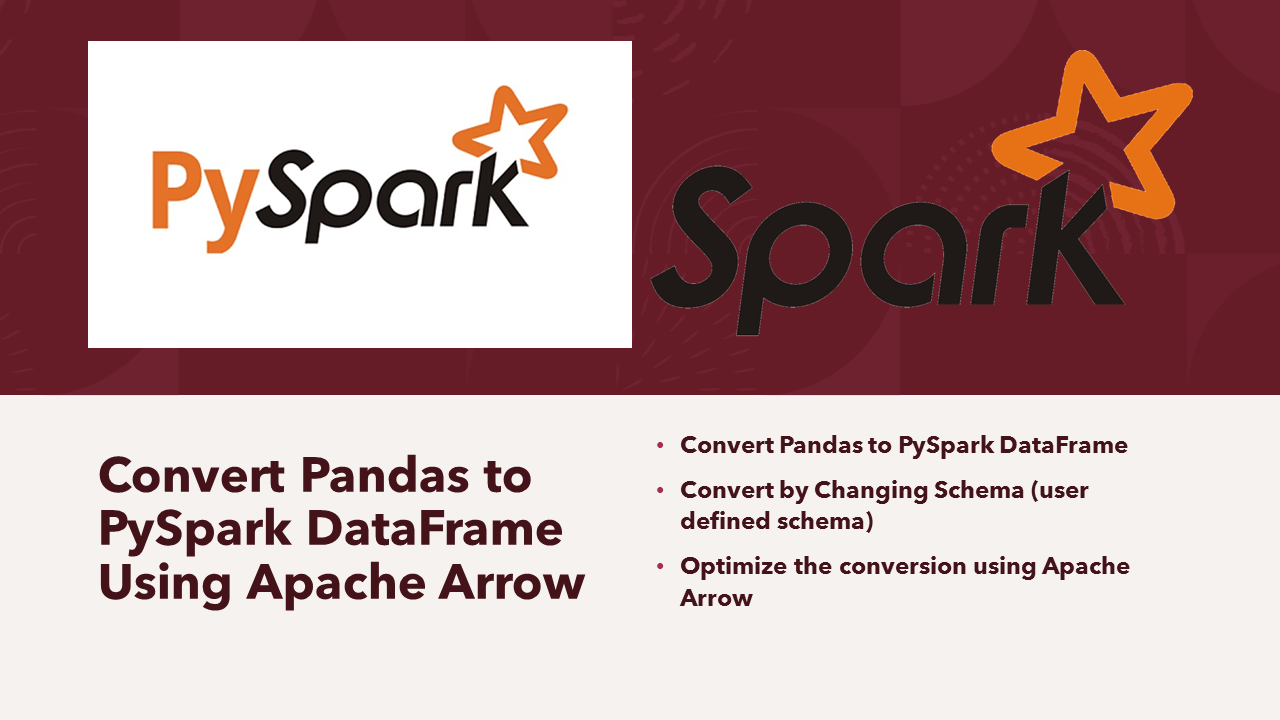# How to Convert Pandas to PySpark DataFrame

While working with a huge dataset Python Pandas DataFrame are not good enough to perform complex transformation operations hence if you have a Spark cluster, it’s better to convert Pandas to PySpark DataFrame, apply the complex transformations on Spark cluster, and convert it back.

In this article, I will explain steps in converting Pandas to PySpark DataFrame and how to Optimize the Pandas to PySpark DataFrame Conversion by enabling Apache Arrow.

## 1. Create Pandas DataFrame

In order to convert Pandas to PySpark DataFrame first, let’s create Pandas DataFrame with some test data. In order to use pandas you have to import it first using `import pandas as pd`

``````
import pandas as pd
data = [['Scott', 50], ['Jeff', 45], ['Thomas', 54],['Ann',34]]

# Create the pandas DataFrame
pandasDF = pd.DataFrame(data, columns = ['Name', 'Age'])

# print dataframe.
print(pandasDF)

# Prints below Pandas DataFrame
Name  Age
0   Scott   50
1    Jeff   45
2  Thomas   54
3     Ann   34
``````

Operations on Pyspark run faster than Python Pandas due to its distributed nature and parallel execution on multiple cores and machines. In other words, pandas run operations on a single node whereas PySpark runs on multiple machines. If you are working on a Machine Learning application where you are dealing with larger datasets it’s a good option to consider PySpark. PySpark processes operations many times faster than pandas.

## 2. Convert Pandas to PySpark (Spark) DataFrame

Spark provides a `createDataFrame(pandas_dataframe)` method to convert Pandas to Spark DataFrame, Spark by default infers the schema based on the Pandas data types to PySpark data types.

``````
from pyspark.sql import SparkSession
#Create PySpark SparkSession
spark = SparkSession.builder \
.master("local") \
.appName("SparkByExamples.com") \
.getOrCreate()
#Create PySpark DataFrame from Pandas
sparkDF=spark.createDataFrame(pandasDF)
sparkDF.printSchema()
sparkDF.show()

#Outputs below schema & DataFrame

root
|-- Name: string (nullable = true)
|-- Age: long (nullable = true)

+------+---+
|  Name|Age|
+------+---+
| Scott| 50|
|  Jeff| 45|
|Thomas| 54|
|   Ann| 34|
+------+---+
``````

If you want all data types to String use `spark.createDataFrame(pandasDF.astype(str))`.

## 3. Change Column Names & DataTypes while Converting

If you wanted to change the schema (column name & data type) while converting Pandas to PySpark DataFrame, create a PySpark Schema using StructType and use it for the schema.

``````
from pyspark.sql.types import StructType,StructField, StringType, IntegerType
#Create User defined Custom Schema using StructType
mySchema = StructType([ StructField("First Name", StringType(), True)\
,StructField("Age", IntegerType(), True)])

#Create DataFrame by changing schema
sparkDF2 = spark.createDataFrame(pandasDF,schema=mySchema)
sparkDF2.printSchema()
sparkDF2.show()

#Outputs below schema & DataFrame

root
|-- First Name: string (nullable = true)
|-- Age: integer (nullable = true)

+----------+---+
|First Name|Age|
+----------+---+
|     Scott| 50|
|      Jeff| 45|
|    Thomas| 54|
|       Ann| 34|
+----------+---+
``````

## 4. Use Apache Arrow to Convert Pandas to Spark DataFrame

Apache Spark uses Apache Arrow which is an in-memory columnar format to transfer the data between Python and JVM. You need to enable to use Arrow as this is disabled by default and have Apache Arrow (PyArrow) install on all Spark cluster nodes using `pip install pyspark[sql]` or by directly downloading from Apache Arrow for Python.

``````
spark.conf.set("spark.sql.execution.arrow.enabled","true")
sparkDF=spark.createDataFrame(pandasDF)
sparkDF.printSchema()
sparkDF.show()
``````

You need to have Spark compatible Apache Arrow installed to use the above statement, In case if you have not installed Apache Arrow you get the below error.

``````
\apps\Anaconda3\lib\site-packages\pyspark\sql\pandas\conversion.py:289: UserWarning: createDataFrame attempted Arrow optimization because 'spark.sql.execution.arrow.pyspark.enabled' is set to true; however, failed by the reason below:
Attempting non-optimization as 'spark.sql.execution.arrow.pyspark.fallback.enabled' is set to true.
``````

When an error occurs, Spark automatically fallback to non-Arrow optimization implementation, this can be controlled by `spark.sql.execution.arrow.pyspark.fallback.enabled`.

``````
spark.conf.set("spark.sql.execution.arrow.pyspark.fallback.enabled","true")
``````

Note: Apache Arrow currently support all Spark SQL data types except `MapType``ArrayType` of `TimestampType`, and nested `<a href="https://sparkbyexamples.com/pyspark/pyspark-structtype-and-structfield/">StructType</a>`.

## 5. Complete Example of Convert Pandas to Spark Dataframe

Belo is complete example to convert Pandas to PySpark DataFrame.

``````
import pandas as pd
data = [['Scott', 50], ['Jeff', 45], ['Thomas', 54],['Ann',34]]

# Create the pandas DataFrame
pandasDF = pd.DataFrame(data, columns = ['Name', 'Age'])

# print dataframe.
print(pandasDF)

from pyspark.sql import SparkSession

spark = SparkSession.builder \
.master("local") \
.appName("SparkByExamples.com") \
.getOrCreate()

sparkDF=spark.createDataFrame(pandasDF)
sparkDF.printSchema()
sparkDF.show()

#sparkDF=spark.createDataFrame(pandasDF.astype(str))
from pyspark.sql.types import StructType,StructField, StringType, IntegerType
mySchema = StructType([ StructField("First Name", StringType(), True)\
,StructField("Age", IntegerType(), True)])

sparkDF2 = spark.createDataFrame(pandasDF,schema=mySchema)
sparkDF2.printSchema()
sparkDF2.show()

# Enable Apache Arrow to convert Pandas to PySpark DataFrame
spark.conf.set("spark.sql.execution.arrow.enabled","true")
sparkDF2=spark.createDataFrame(pandasDF)
sparkDF2.printSchema()
sparkDF2.show()

#Convert PySpark DataFrame to Pandas
pandasDF2=sparkDF2.select("*").toPandas
print(pandasDF2)

``````

### Conclusion

In this article, you have learned how easy to convert Pandas to Spark DataFrame and optimize the conversion using Apache Arrow (in-memory columnar format)

Happy Learning!!

### NNK

SparkByExamples.com is a Big Data and Spark examples community page, all examples are simple and easy to understand and well tested in our development environment Read more ..